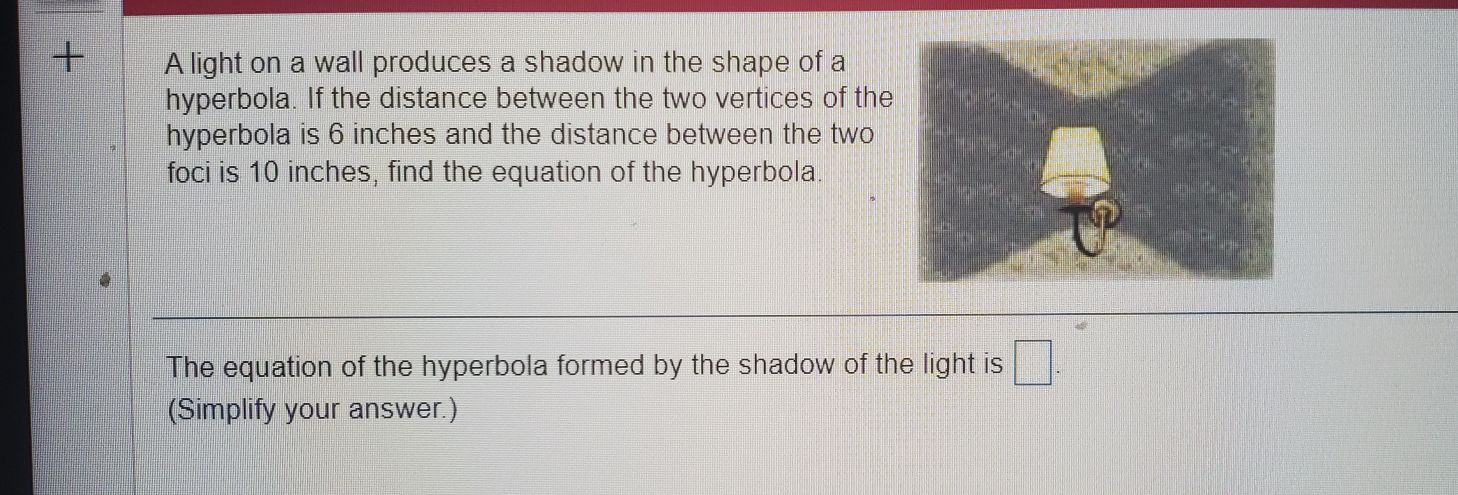### ¿Todavía tienes preguntas de matemáticas?

Pregunte a nuestros tutores expertos
Algebra
PreguntaA light on a wall produces a shadow in the shape of a hyperbola. If the distance between the two vertices of the hyperbola is $$6$$ inches and the distance between the two foci is $$10$$ inches, find the equation of the hyperbola. The equation of the hyperbola formed by the shadow of the light is $$\square$$ . (Simplify your answer.)

$$\frac{x^2}{9} - \frac{y^2}{16} = 1$$